Smallest number divided to get perfect square

Chapter 5 Class 8 Squares and Square Roots
Concept wise

Let’s do this by examples

#### Finds the smallest number divided to 32 to get a perfect square.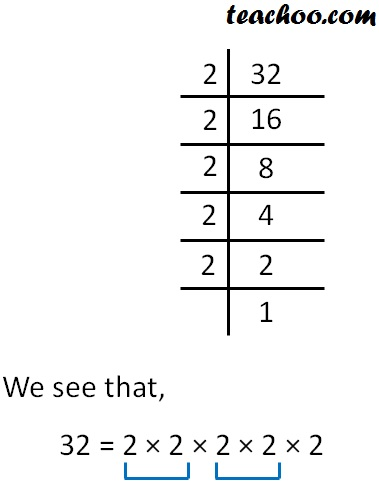Since 2 does not occur in pairs,

we divide by 2 to make it a pair

So, our number becomes

32 × 1/2 = 2 × 2 × 2 × 2 × 2 × 1/2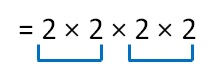Now, it becomes a perfect square.

So, we divide 32 by 2 to make it a perfect square

#### Finds the smallest number divided to 90 to get a perfect square.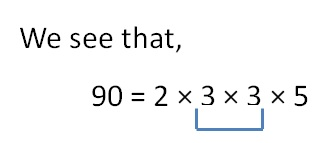Here,

2 & 5 do not occur in pairs

So, we divide by 2 and 5 to make pairs

So, our number becomes

90 × 1/2 × 1/ 5 = 2 × 3 × 3 × 5 × 1/2 × 1/5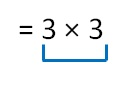Now, it becomes a perfect square.

So, we divide 90 by 2 × 5

i.e. 10  to make it a perfect square

#### Finds the smallest number divided to 120 to get a perfect square.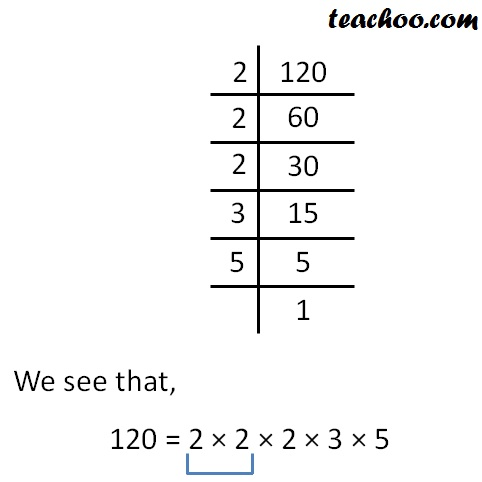Here,

2, 3 & 5 do not occur in pairs

So, we divide by 2, 3 and 5 to make pairs

So, our number becomes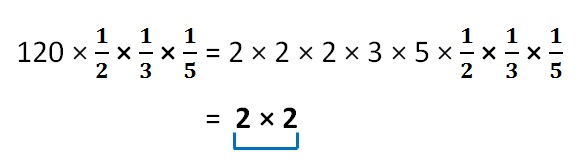Now, it becomes a perfect square.

So, we divide 120 by 2 × 3 × 5

i.e. 30  to make it a perfect square

Learn in your speed, with individual attention - Teachoo Maths 1-on-1 Class